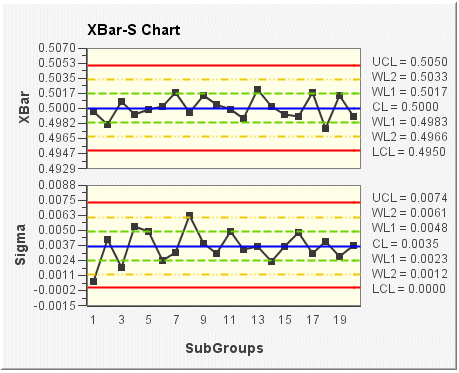# XBar-S chart

The XBar-S chart displays two charts: a control chart for subgroup means (XBar chart) and a control chart for subgroup standard deviations (Sigma chart) that are drawn in the same graph window in the upper and lower halves of the screen respectively. Standard deviation uses each individual reading to calculate variability; therefore, Sigma is a more efficient indicator of process variability than Range, especially with larger sample sizes. This composite chart helps to track both the process level and process variation at the same time, as well as to detect the presence of special causes.

In the following XBar-S chart example, the subgroup size for the sample data is 5. In the top chart (XBar), the mean (average) of each subgroup is plotted. In the bottom chart (Sigma), the sigma (standard deviation) of each subgroup is plotted. The control limits (shown on the chart as UCL, CL, LCL, WL1, and WL2) are set according to the data and a sigma limit of 3.